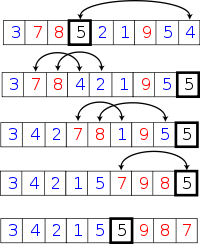# 查找第K小的元素1. import random
2.
3. def partition(arr, left, right, pivot):
4.     v = arr[pivot]
5.     arr[pivot], arr[right-1] = arr[right-1], arr[pivot]
6.     index = left
7.     for i in xrange(left, right):
8.         if arr[i] <= v:
9.             arr[i], arr[index] = arr[index], arr[i]
10.             index += 1
11.     return index-1
12.
13. def select(arr, left, right, k):
14.     while right - left > 1:
15.         index = partition(arr, left, right, random.randint(left, right-1))
16.         dist = index - left + 1
17.         if dist == k:
18.             return arr[index]
19.         if dist < k:
20.             k -= dist
21.             left = index + 1
22.         else:
23.             right = index
24.     return arr[left]

T(n)<=T(n/5)+T(7/10*n)+O(n)<=c*n*(1+9/10+(9/10)^2....) 所以T(n)=O(n)

1. import heapq
2.
3. def partition(arr, left, right, pivot):
4.     v = arr[pivot]
5.     arr[pivot], arr[right-1] = arr[right-1], arr[pivot]
6.     index = left
7.     for i in xrange(left, right):
8.         if arr[i] <= v:
9.             arr[i], arr[index] = arr[index], arr[i]
10.             index += 1
11.     return index-1
12.
13. def select_heap(arr, left, right, k):
14.     tmp = [(arr[i], i) for i in range(left, right)]
15.     heapq.heapify(tmp)
16.     [heapq.heappop(tmp) for i in xrange(k-1)]
17.     return heapq.heappop(tmp)
18.
19. def median(arr, left, right):
20.     num = (right - left - 1) / 5
21.     for i in xrange(num+1):
22.         sub_left = left + i*5
23.         sub_right = sub_left + 5
24.         if sub_right > right:
25.             sub_right = right
26.         m_index = select_heap(arr, sub_left, sub_right, (sub_right-sub_left)/2)
27.         arr[left+i], arr[m_index] = arr[m_index], arr[left+i]
28.     return select(arr, left, left+num+1, (num+1)/2)
29.
30. def select(arr, left, right, k):
31.     while right - left > 1:
32.         pivot = median(arr, left, right)
33.         index = partition(arr, left, right, pivot)
34.         dist = index - left + 1
35.         if dist == k:
36.             return (arr[index], index)
37.         if dist < k:
38.             k -= dist
39.             left = index + 1
40.         else:
41.             right = index
42.     return (arr[left], left)

### 《查找第K小的元素》有 11 条评论

1.orbbyrp | #1

MS算法导论上有……好像直接拿概率的pivot破要简单……

•isnowfy

@orbbyrp: 不是啊，我这里不仅是平均复杂度是O(n)，在最坏情况下也是O(n)，如果是概率的pivot，最坏是O(n^2)

2.wyncat | #2

哈哈，貌似算法课上学了这个的。感觉没白上课。。。。

•isnowfy

@wyncat: 赞算法课！

3.TeeKee | #3

受教了，请问用的是什么语言实现的？因为自己一直用C++，还真没见过这个。

•isnowfy

@TeeKee: python实现的

4.William | #4

感觉是错的

5.William | #5

找中位数的最坏情况本身就是O(n2)的

•isnowfy

@William: 最坏情况是O(n)不管是5个数的中位数还是n/5个数的中位数都是O(n)，如果实在不理解，请仔细阅读文章中wiki的链接